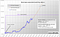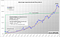# Updated Bitcoin Forecast until June

Concrete bitcoin formula for the hyper-exponential growth forecast

# Introduction

As I detailed in my story “A Little Math and a Bitcoin Forecast” , the current bitcoin price follows a mathematical formula.
Now that enough values have been added to the formula, I was able to update it accordingly and adjust the parameters.
Contrary to what I initially thought, the current price does not follow a double exponential function, but it’s a triple one.

# The Formula

The general form of the formula is:

USD/BTC = BTC(SF)@halving * e ^ (at (bt² + 1))

BTC(SF)@halving : is the initial Stock-To-Flow Bitcoin price at the time of the halving
a: is the factor that determines the initial growth at halving
b: is the factor that determines the growth of the growth itself after halving
t: is the time span between the halving (t = 0) and the date for which the value is to be determined.

The factor b is a quadratic factor, so the whole formula becomes cubic with respect to time. If you multiply it out, you get (when replacing ab by b):

USD/BTC ≈ BTC(SF)@halving * e ^ (at + bt³)

For the current phase after the halving at 11.05.2020, taking into account the real values:

USD/BTC ≈ 9100 * e ^ (0.81t + 1.9t³)

It may be hard to believe, but this simple formula describes the price trend of the Bitcoin after halving.
For the statisticians among you, here is the correlation coefficient for the equation above:
r = 0.9767 [from 11.05.2020 until 22.03.2021]Bitcoin hyper-exponential growth 2021

In the chart you can see how well it reflects the actual course until now.
A regularly updated chart can be found here:

To show how well this formula is also suitable for the bitcoin course of 2016/17, here is the concrete formula for this period:

USD/BTC ≈ 510 * e ^ (0.81t + 0.7t³)
r = 0.97
[from 9.5.2016 until 30.12.2017]

As can be seen, the initial growth at halving in 2020 is just as large as the growth at halving in 2016.
The only difference is that the factor of growth in 2017 is much smaller, since the increase is also much flatter than that of 2021.Bitcoin hyper-exponential growth phase 2017

To check the formulas, we can take a point in time just one year after the halving:

2017/07/09 : USD/BTC ≈ 510 * e ^ 1.51 ≈ \$2,309
and
2021/05/11 : USD/BTC ≈ 9100 * e ^ 2.71 ≈ \$136,766

The first value can be checked very easily. It was \$2,573 at that time, three days later it was \$2,333. That precision is awesome for such a long time period.
The second value for 2021 we have to wait for, but I am very confident that it will be reached as well. If this value is reached, it certainly cannot be dismissed as a coincidence.

# Explanation

First of all, it is important to realize that the Bitcoin price does not increase because people or companies are driving or even manipulating it.
Also, the current economic situation has no influence on the value. No, the price solely follows an intrinsic value, which is described very nicely by the Stock-To-Flow theory for Bitcoin by PlanB .
Almost all the stories you read about the reasons for the rise of bitcoin prices belong in a storybook. Otherwise one could not simply put the value into a formula. I think this will be always ignored.
The Bitcoin price is the result of a stable economic equilibrium . Any momentary deviation of the value from this equilibrium leads very quickly back to the actual intrinsic value. This can be shown very nicely by the example of TESLA’s entry into the Bitcoin market with 1.5 billion dollars. Exactly on 8.2.2021 the value rises by the purchase of this quantity on approx. 20%. However, the value falls back to the calculated value within 20 days (see chart 2021). This is also true for all other large purchases or sales. The value can always only be out of equilibrium for a short period of time and then reliably falls back each time.
This equilibrium changes over time and its time course after a halving is described by my formula.

I think there are three influences on growth after halving:
1. constant consumption of Bitcoins → there’s a whole industry using BTC
2. scarcer supply → the higher the price the less sell.
3. increasing demand → the higher the price the more buy.
1 is repsonsible for the initial growth, 2 & 3 together for the increase in growth. That’s what the equation says.

It will certainly take quite a while for this wisdom to become accepted by all economists. But the beginning has already been made with the Stock-To-Flow theory, which is gaining more and more recognition.

# Forecast

With this formula, the future values can be easily calculated.
If you take the first day of each of the coming months, the result is:

2021/4/1 : BTC/USD ≈ \$72,816
2021/5/1 : BTC/USD ≈ \$117,265
2021/6/1 : BTC/USD ≈ \$207,671

At the beginning of July, the calculated value is \$392,340. This sum is certainly much too high to actually be reached, making a crash in June virtually inevitable. Possibly even before in May.
The inner stock to flow value is about \$85,000 in June according to the formula from PlanB. (I used the formula that predicts the lowest value).
In 2017, bitcoin value reached about three times the stock to flow value (\$20000 and BTC(SF) = \$6500). If there is a similar exaggeration this time, the highest value would be 3x \$85,000 = \$255,000.
However, this is only a rough indication, because it is actually impossible to determine the exact date of the crash. The probability of a crash just increases with the bitcoin price.
The crash is the result of a chain reaction when the first Bitcoin whale sells its coins and the price falls accordingly. The euphoria then suddenly turns into panic.

But what happens after the crash? Fortunately, the Bitcoin value trend of 2018 gives an answer. The price will most likely fall at most to the
Stock to Flow value, so it will end up above \$85,000. Moreover, it will then fluctuate a lot for a while, as many people still believe in a further increase of the price.
After all, the bitcoin value has to follow the stock to flow value, otherwise this theory would not be valid. But it has been doing so since the beginning of the Bitcoin market in 2010, which should suffice as an argument.

# Conclusion

Although it may be hard to believe, there is actually a Bitcoin formula for long-term growth (S2F theory) and a formula for short-term hyper-exponential growth after halving. You can actually life track now how the bitcoin value aligns with these formulas. Then it should really be clear to everyone that these formulas have their justifications.
Every investor can now draw his own conclusions. Be it to sell his Bitcoins as expensive as possible, or to wait for further cycles in order to benefit in 4 years to profit again from the rise after halving. Should the popularity of the Bitcoin continue to rise until then, then there will most likely be another strong growth beginning after the next halving.

As always, please note the following:
This is only my personal opinion and in no way to be taken as investment advice. The predictions made here are purely subjective and do not have to be correct in any way.

# References

 A Little Math and a Bitcoin Forecast, by Pisu
https://medium.com/coinmonks/a-little-math-and-a-bitcoin-forecast-bcaddc17d252

 Bitcoin Forecast Chart, by Pisu
https://www.pisu666.de

 Modeling Bitcoin Value with Scarcity, by PlanB
https://medium.com/@100trillionUSD/modeling-bitcoins-value-with-scarcity-91fa0fc03e25

 Economic Equilibrium (Wikipedia)
https://en.wikipedia.org/wiki/Economic_equilibrium

I’m just a guy with a computer that likes playing around with numbers

## More from Pisu

I’m just a guy with a computer that likes playing around with numbers